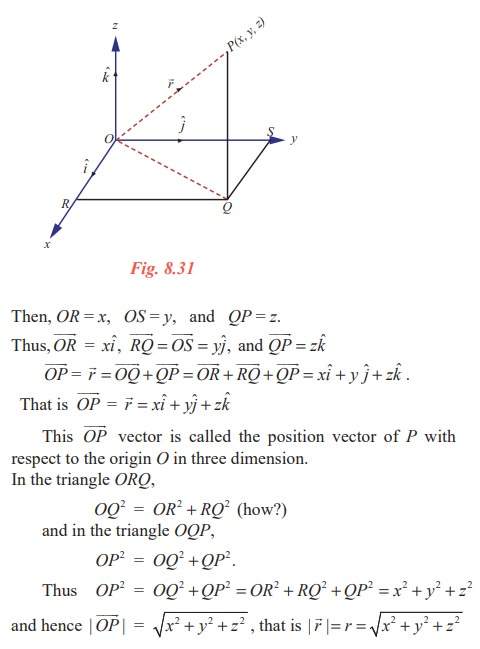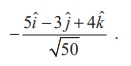Home | | Maths 11th std | Resolution of Vectors

# Resolution of Vectors

Resolution of a vector can be done for any finite dimension. But we will discuss only in two and three dimensions. Let us start with two dimension.

Resolution of Vectors

Resolution of a vector can be done for any finite dimension. But we will discuss only in two and three dimensions. Let us start with two dimension.

## Resolution of a vector in two dimension

Theorem 8.5

Let iˆ and ˆj be the unit vectors along the positive x-axis and the y-axis having initial point at the origin O. Nowis the position vector of any point P in the plane. Thencan be uniquely written as= xiˆ + y jˆ for some real numbers x and y. FurtherProof

Let (x, y) be the coordinates of the point P. Let L and M be the foots of the perpendiculars drawn from P to the x and y axes. ThenTo prove the uniqueness, let x1iˆ + y1 ˆj and x2 iˆ + y2 ˆj be two representations of the same point P. Then

x1iˆ + y1jˆ = x2iˆ + y2jˆ .

This implies that

(x1 − x2 )iˆ − ( y2− y1 ) ˆj =x1 − x2 = 0, y2 − y1 = 0.

In other words x1 = x2  and y1 = y2  and hence the uniqueness follows.

In the triangle OLP, OP2 = OL2 + LP2 ;

hence ||=  √[ x2 + y2 ].That is,Observe that if iˆ + jˆ are the unit vectors in the postive directions of x and y axes, then the  iˆ and jˆ position vector of the point (6,4) can be written as 6iˆ + 4 jˆ  and this is the only way of writing it.

Result 8.8

Ifandare two non-collinear vectors in a plane, then any vector in the plane can be written as the linear combination ofandin a unique way. That is, any vector in the plane is of the form l+ mfor some scalars l and m.

ProofNote 8.2

Further if three non collinear vectors are coplanar then any one of the vector can be written as a linear combination of other two. Note that the converse is also true.

Result 8.9

If,andare three non-coplanar vectors in the space, then any vector in the space can be written as l+ m+ nin a unique way for some scalars l, m and n.

Definition 8.15

Let iˆ and ˆj be the unit vectors in the positive directions of x and y axes respectively. Letbe any vector in the plane. Then= xiˆ + y ˆj for some real numbers x and y. Here xiˆ and y ˆj are called the rectangular components ofalong the x and y axes respectively in two dimension.

What we discussed so far can be discussed in the three dimensional space also.

## Resolution of a vector in three dimension

Theorem 8.6

Let iˆ, ˆj and kˆ be the unit vectors in the direction of postive x, y and z axes respectively having initial point at the origin O. Letbe the position vector of any point P in the space. Thencan be uniquely written as= xiˆ + y ˆj + zkˆ for some real numbers x, y and z. Further || = √ [ x 2+ y 2 + z2 ].

Proof

Let (x, y, z) be the coordinates of the point P. Let Q be the foot of the perpendicular drawn from P to the xy-plane. Let R and S be the foots of the perpendiculars drawn from Q to the x and y axes respectively. Let=.

Then, OR = x, OS = y, and QP = z. z### Components of vector joining two points

Let us find the components of the vector joining the point  (x1 , y1 ) to (x2 , y2 ).

Let A and B be the points (x1 , y1 ) and (x2 , y2 ). Let P be the point (x2x1 , y2y1 ). Then=.

The components ofare  (x2x1 )iˆ and ( y2y ) jˆ. Hence the components ofin the directions of x and y axes are (x2 − x1 )iˆ ( y2 − y1 ) jˆ.

Similarly if A and B are the points (x1 , y1 , z1 ) and (x2 , y2 , z2 ), then the components ofin the directions of x, y and z axes are (x2 − x1)iˆ ( y2y1 ) jˆ (z2z1 )kˆ .

## Matrix representation of a vector

A vector with three components can be visualised as either a row or column matrix as [x, y, z] orrespectively.

Thus any vector= a1iˆ + a2 jˆ + a3kˆ can be obtained fromHence addition of vectors and multiplication of a vector by a scalar can be defined as follows.k= ka1iˆ + ka2 ˆj + ka3 kˆ

For k R, k > 1 yields magnification, 0 < k < 1 yields contraction of a vector and k = 0 yields a zero vector= 0iˆ + 0 ˆj + 0kˆ =.

Result 8.10

Using the commutative, associative properties of vector addition and the distributive property of the scalar multiplication we can prove the following.Example 8.4

Find a unit vector along the direction of the vector 5iˆ − 3 ˆj + 4kˆ.

Note 8.3

Now we have another unit vector parallel to 5iˆ − 3 ˆj + 4kˆ in the opposite direction. That is,Tags : Definition, Theorem, Solved Example Problems | Mathematics , 11th Mathematics : UNIT 8 : Vector Algebra I
Study Material, Lecturing Notes, Assignment, Reference, Wiki description explanation, brief detail
11th Mathematics : UNIT 8 : Vector Algebra I : Resolution of Vectors | Definition, Theorem, Solved Example Problems | Mathematics